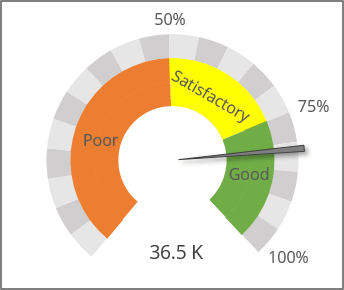# Speedometer chart

Excel 365
A speedometer, dial, or gauge chart displays a single value (usually displayed as an arrow) on a colored or sectored dial. The speedometer dial can be a half or a whole circle (the simplest option) or a sector located symmetrically vertically: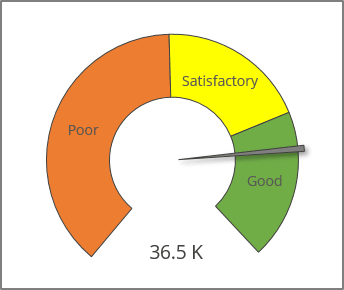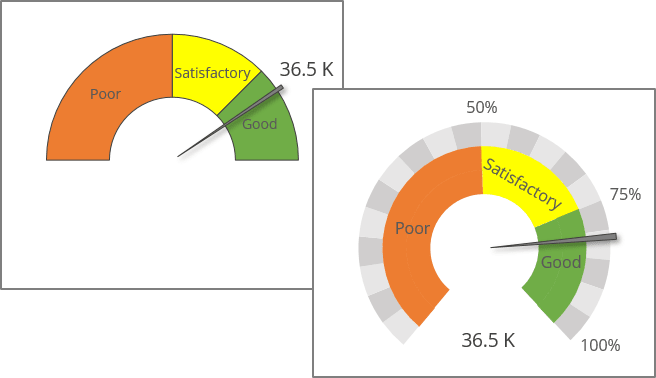In Excel, you can use pie and donut (doughnut) chart types to create a speedometer chart. See also the simplest variant in how to create a gauge chart.

To create a speedometer chart like the one above, do the following:

## Prepare data ranges

1.   To create a speedometer graph, you need several different data ranges:

• The actual or current value:

For example:

• Cell B2 contains the actual value that is displayed as an arrow,
• Cell C2 contains the total amount and the Goal value.
• To display an arrow, calculate the following data: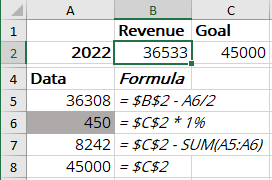• Cell A5 contains the current or actual value minus half of the data used for the arrow if you prefer to display a glancing arrow:

= <value> - <arrow> / 2

• Cell A6 contains the data used to display an arrow:
• 1 if you have small amounts or it is enough to display an arrow as a simple line,
• 1% of the Goal value if you prefer to see a glancing arrow:

= <Goal> * 1%

• Cell A7 contains the residual value:

= <Goal> - SUM (A5:A6) or = <Goal> - <value> - <arrow> / 2

• Cell A8 contains the value for the invisible section of the chart:
• The value is equal to the Goal value to display the exactly half-cycled speedometer chart like in the first example:

= <Goal>

• Any other value, for example, 30% of the Goal value like in the second example:

= <Goal> * 30%

• The qualitative ranges are displayed as colored zones:

For example, using the  poor ,  satisfactory , and  good  value ranges, which are distributed as follows: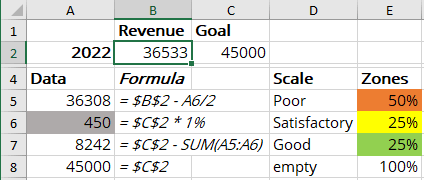The empty value is an invisible value that corresponds to the value in cell A8:

• 100% for the first example,
• 30% for the second example.

## Create a combo chart

2.   Select the data (in this example, A5:A8, E4:E8).

3.   On the Insert tab, in the Charts group, click the Combo button, then choose Create Custom Combo Chart...: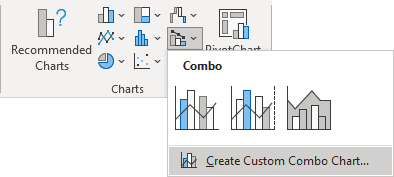In the Insert Chart dialog box:

• For the data series with values (in this example, series Data):
• Choose the Pie chart type,
• Ensure that the Secondary axis checkbox is selected.
• For the second data series with zones (in this example, series Zones), choose the Doughnut chart type: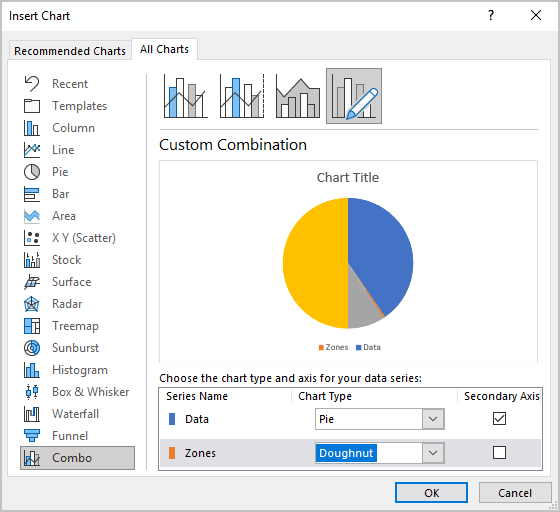## Format chart

4.   Optionally, remove the chart title and the legend.

### Select the Pie data series

5.   Select the Pie data series (in this example, series Data) by doing one of the following:

• Select the chart, then on the Chart Format tab, in the Current Selection group, from the Chart Elements list, select the appropriate data series: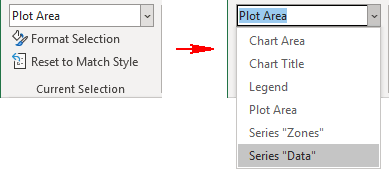• Right-click on the chart plot area, then select Format Plot Area... in the popup menu: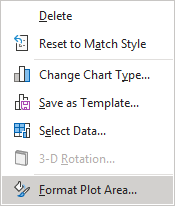On the Format Plot Area pane, click the arrow next to Plot Area Options, then choose the element you need from the dropdown list: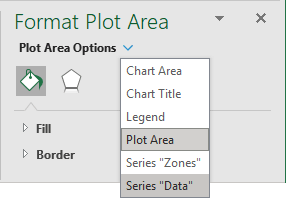### Rotate the Pie data series

6.   On the Format Data Series pane, on the Series Options tab, change the Angle of first slice setting to:

• 270o for the first example: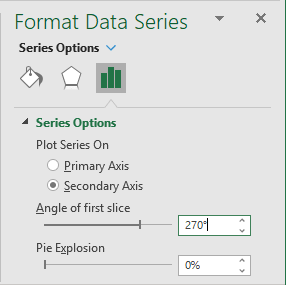• ~222o for the second example:

= (SUM (<Zones>) / 2 + <empty>) / (SUM (<Zones>, <empty>) * 360o)

### Select the Pie data points and format them

7.   Select the data point of the Pie chart data series by doing one of the following:

• Double-click twice on the data point you want to format,
• Hold the Ctrl key and use the arrow buttons to select your desired chart element.

If needed, right-click on the selection and choose Format Data Point... in the popup menu.

8.   On the Format Data Point pane, on the Fill & Line tab:

• For the data points but the data point for the arrow (in the example, A6):
• In the Fill section, select No fill,
• In the Border section, select No Line: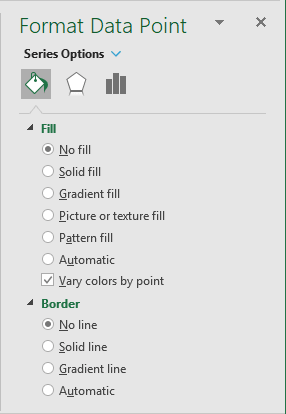• Choose the appropriate filling for the arrow data point (see more about filling in Microsoft Excel).

For example: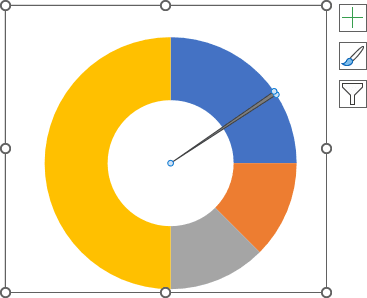### Format the Doughnut data series

9.   Repeat step 4 to select the Doughnut data series (in this example, series Zones).

10.   Repeat step 5 to rotate the second data series: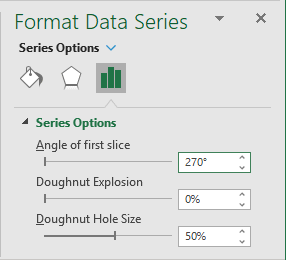11.   Repeat step 6 to open the Format Data Point pane for each data point from the Doughnut data series.

On the Format Data Point pane, on the Fill & Line tab:

• Choose the appropriate filling for the data points but the last one (in the example, E8),
• Hide the last data point:
• In the Fill section, select No fill,
• In the Border section, select No Line.

For example: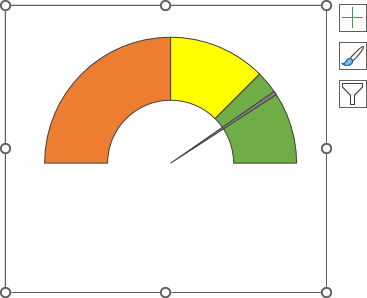You can then make any other adjustments to get the look you desire.

Notes:

1. To make an arrow a little "bigger" than another data on the chart, select that data point, then on the Format Data Point pane, on the Series Options tab, change the Point Explosion setting to 5%: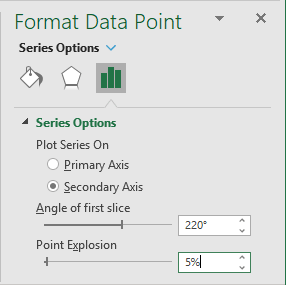2. You can add several data series to your chart with the Doughnut chart type: